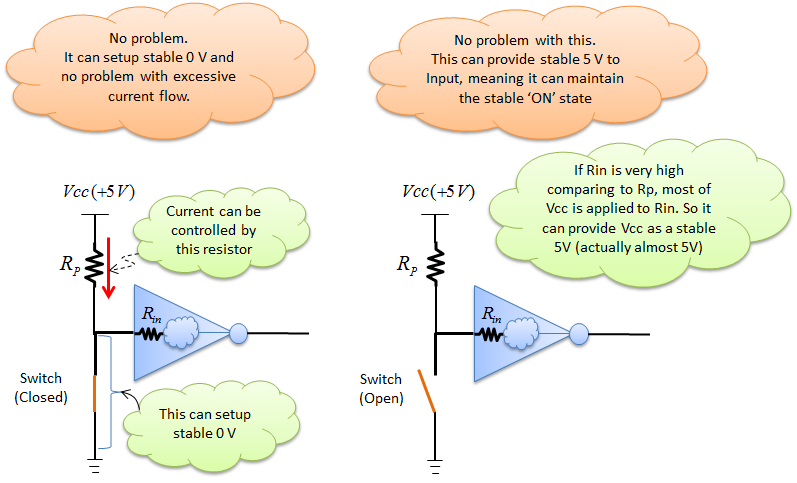Electronics - Pull Up Resistor                                                  Home : www.sharetechnote.com In engineering, there are many words that does not make much sense if you just literally translate it (like dictionary). The term 'Pull-up Resistor' is one of them (it didn't make much sense at least to me). I asked myself "What ? Pull up what ?". I also googled a lot trying to find where this term came from, but failed. In some context, it sounds like 'Pull up means 'Pulling up the input voltage to High state'" but not so sure to me.   So instead of trying to make sense out of the word itself, let's look into why we need this kind of special resistor and how it works.   In many case, we explain a digital circuit with ideal switch logic. It is conceptually simple and easy to understand, but it can be misleading when you apply it to real circuit.   Let's follow a simple example step by step. Just take a look at the illustraions and give some thought to the question and comments that I put on the illustration.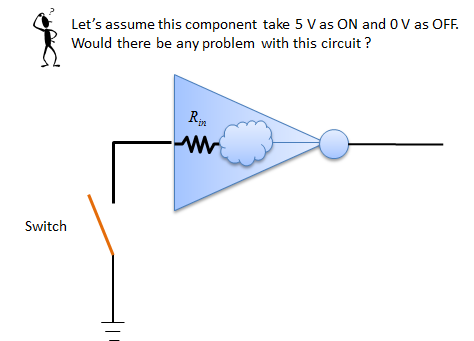In this circuit, there wouldn't be much problem when the switch is closed setting up '0' state to the input pin, but there would be issues when trying to set up '1' state by openning the switch. See the following illustration.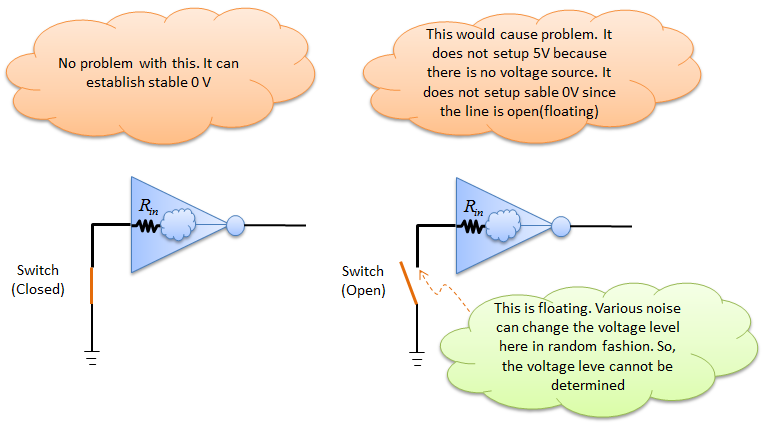Trying to solve the issues shown above, we connected a voltage source as follows. Would this solve all the problem ?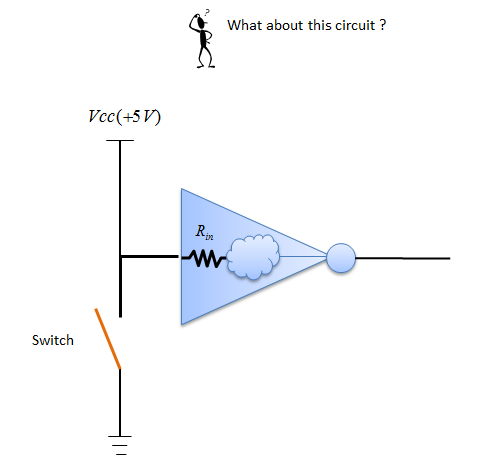If you just think of the digital logics (1 and 0) like conceptual switch, there seems to be no problem. But if you implement the circuit like this, you may have some practical problem as described in the following illustration.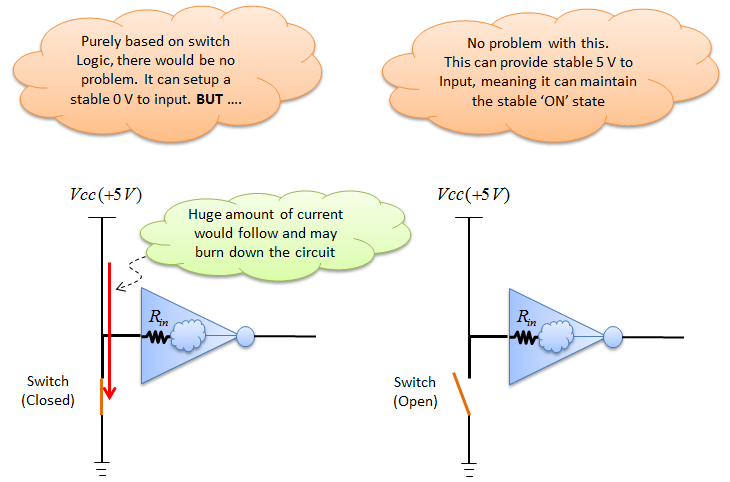To resolve this practical problem, they put a resistor as follows.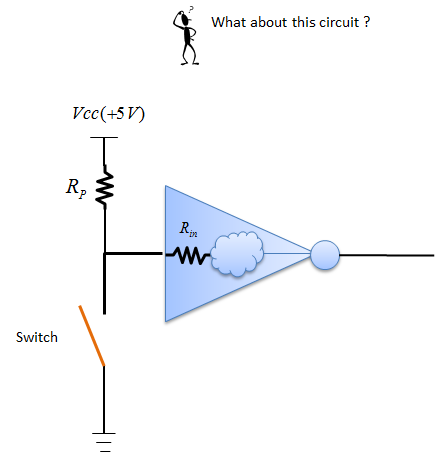According to the following illustration, it seems that this simple resistor solves the problem that we had. This little tiny resitor is called 'Pull-Up' resistor.